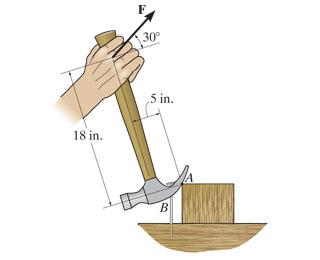# Moment about a point of a hammer

## Homework StatementIn order to pull out the nail at B, the force F exerted on the handle of the hammer must produce a clockwise moment of 590 lb⋅in about point A.(Figure 1)

Determine the required magnitude of force F.

## The Attempt at a Solution

M=590lb*in

-590= -Fcos(30)(18)-Fsin(30)(5)

I'm not sure how to deal with F. I know it's simple if given the force to determine the moment but I'm a bit stumped trying to go the other way.

haruspex
Homework Helper
Gold Member
2020 Award
590lb*in

-590= -Fcos(30)(18)-Fsin(30)(5)

I'm not sure how to deal with F. I know it's simple if given the force to determine the moment but I'm a bit stumped trying to go the other way.
How would you solve 3=x+2x?

So, F=72.9431?

haruspex
Homework Helper
Gold Member
2020 Award
So, F=72.9431?

andrewkirk
Homework Helper
Gold Member
Let ##X## be the point where the force is applied to the handle.

The angle you need to use is not 30 degrees but the angle ##\alpha## between the force vector and the perpendicular to the line segment ##\overline{XA}##. You can calculate that angle using geometry, from the information given.

The Torque (moment) will be the force multiplied by ##\cos\alpha## and the length of ##\overline{XA}##.

By the way, that's one weird hammer! Based on the drawing and the dimensions given, the claw is about eight inches long! That's a gemmy, not a hammer. The drawing is not to scale. The 18 in length is not 18/5 times the 5 inch length. That's why the hammer doesn't look weird.

Sorry, -590=-F18cos(30)-5Fsin30)=32.6175

haruspex
Homework Helper
Gold Member
2020 Award
Sorry, -590=-F18cos(30)-5Fsin30)=32.6175
Yes.

haruspex
Homework Helper
Gold Member
2020 Award
The angle you need to use is not 30 degrees but the angle αα\alpha between the force vector and the perpendicular to the line segment ¯¯¯¯¯¯¯¯¯XAXA¯\overline{XA}. You can calculate that angle using geometry, from the information given.
the method Robb used is valid, and quite efficient.

•Robb
Yes.
Dang, nothing like making a mountain out of a mole hill!

andrewkirk